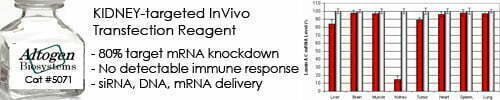••••Shop Products

# Body Surface Area Calculator

A Body Surface Area (BSA) calculator is a tool that calculates the surface area of a person’s body based on their height and weight. BSA is a measure of the total surface area of a person’s skin and is commonly used in medicine to calculate drug dosages, assess burns and other skin injuries, and estimate the energy requirements of a patient.

The most commonly used formula for calculating BSA is the Du Bois formula:

BSA = 0.20247 x Height (m)^0.725 x Weight (kg)^0.425

Where:

• Height is the person’s height in meters
• Weight is the person’s weight in kilograms

To use a BSA calculator, you need to input the values of height and weight, and the calculator will calculate the corresponding BSA for you. The resulting BSA value can then be used in medical calculations to determine appropriate drug dosages, nutritional needs, or other clinical applications.

Other formulas are also used to calculate BSA, such as the Mosteller formula, which is based on a person’s height and weight but uses a different exponent for each variable. The choice of formula may depend on the specific clinical application and the population being studied.

Body Surface Area Calculator